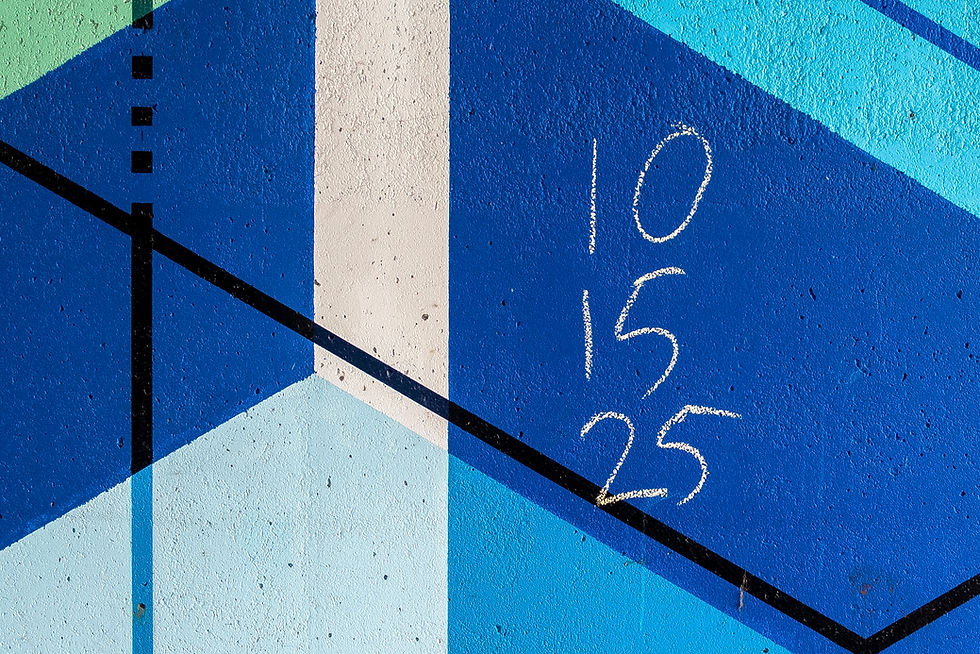top of page
Search

# How Our Math Program Works

Mathematics is a fascinating subject that has been studied for thousands of years. It is the foundation of many disciplines, including physics, engineering, economics, and computer science, to name a few. Mathematics is also a subject that can be enjoyed by people of all ages and backgrounds, regardless of whether they are pursuing a career in a math-related field or simply interested in learning for fun.There are many different levels of math that one can explore, depending on their interests and goals. At the most basic level, there is arithmetic, which involves the manipulation of numbers and the four basic operations: addition, subtraction, multiplication, and division. This is a fundamental skill that is essential for everyday life, from balancing a checkbook to calculating a tip at a restaurant.

Moving up from arithmetic, there is algebra, which introduces the concept of variables and the use of letters to represent unknown quantities. Algebra is used to solve equations, factor polynomials, and study the properties of functions, among other things. This is a crucial foundation for higher-level math, such as calculus and linear algebra.

Calculus is the study of change and rates of change, and is used to solve problems in physics, engineering, and economics, among other fields. Linear algebra is the study of vector spaces and linear transformations, and is used in computer graphics, data analysis, and machine learning, to name a few examples.

Our Math Program is subdivided into the following sections:At the K-G6 MPM level, we focus primarily on teaching kids the basics of numbers and arithmetic. Numbers are the building blocks of math. We will teach them all the basic operations of numbers as well as how to use measurements and units. From there, we will cover algebraic equations, fractions and decimals, probability, and statistics.

We also offer one-on-one tutoring for the G3-G5 level as well as the G6-G7 level. At the G3-G5 level, we focus on basic geometry. We teach them how to measure angles and lengths as well as manipulate fractions and decimals. We expand on numerical concepts and operations and dive a bit deeper into arithmetic operations.

At the G6-G7 level, we expand upon the earlier levels by going into rational numbers, more advanced algebraic equations, as well as basic graphing. We will teach kids 2D and 3D geometry, including how to find the distance, angles, midpoints, and areas between triangles. We will also go a bit more advanced into probability and statistics, expanding on knowledge from previous levels.

For G8-G10, they will learn how to graph equations and manipulate them. They will learn more advanced algebraic equations as well as linear and non-linear relationships. They will explore practical applications and will be able to apply their learning to real world problems in both economics and statistics.

In the final level fit for Grades 11 and 12, they will learn Calculus. They will learn polynomial equations and how to graph them. They will learn to analyze these graphs. They will explore trigonometric functions as well as exponential and logarithmic equations. Then, they will proceed towards learning differential equations, integrals, and derivatives as well as learn practical applications of calculus.

No matter what level of math one is studying, there is always something new to discover and explore. Math is a subject that can be challenging, but it is also incredibly rewarding. It can help us solve complex problems, make sense of the world around us, and even uncover new ideas and theories. So whether you are just starting out with arithmetic or diving into the deep end of calculus, embrace the challenge and enjoy the journey!